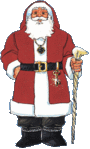Speaker: Alessandro Paolini (Birmingham)

Title: Reduction of Characters of Sylow $$p$$-Subgroups of Finite Groups of Lie Type

Abstract:

Let $$q$$ be a power of a prime $$p$$. We denote by $$G$$ a finite group of Lie type which is split over $$F_q$$, and by $$U = U(G)$$ a Sylow $$p$$-subgroup of $$G$$. We are interested in a parametrization of the irreducible characters of $$U$$, which is "uniform" over good primes $$p$$. This is motivated by the determination of the generic character tables for $$U$$, with a view towards the determination of decomposition numbers of $$G$$. I will first present some recent results of Himstedt, Le and Magaard, that hold when the prime $$p$$ is not very bad for $$G$$, about the parametrization of the irreducible characters of $$U(D_4(q))$$. I will then show how the ideas in this work lead to a parametrization of the irreducible characters of any $$U$$, via a reduction procedure by successive subquotients of $$U$$. This is obtained in a joint work with Goodwin, Le and Magaard when $$U$$ is a Sylow $$p$$-subgroup of any group of Lie type of rank at most 4. In particular, for $$U(F_4(q))$$, the parameterization is "uniform" over all primes $$p > 3$$, where all character degrees are of the form $$q^d$$ for some integer $$d$$, but this does not happen for the bad prime $$p = 3$$.